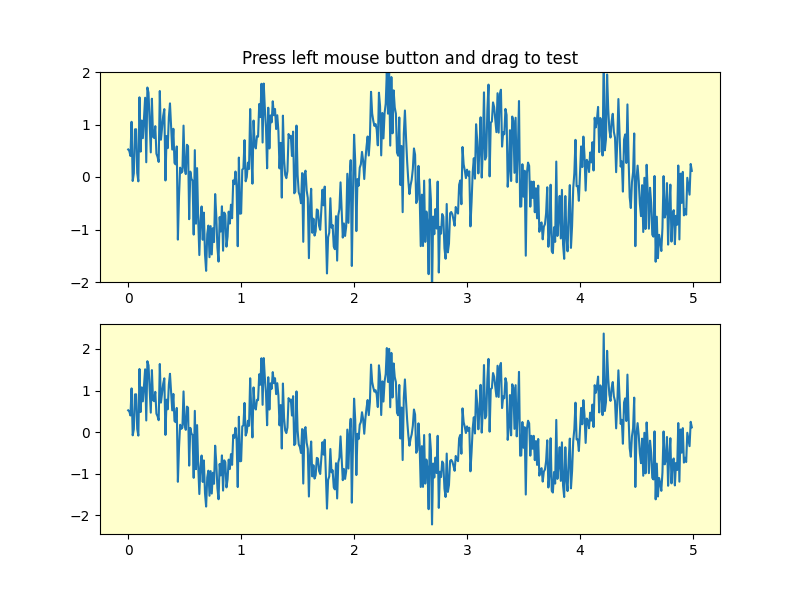Span Selector¶

The SpanSelector is a mouse widget to select a xmin/xmax range and plot the detail view of the selected region in the lower axes

import numpy as np
import matplotlib.pyplot as plt
from matplotlib.widgets import SpanSelector

# Fixing random state for reproducibility
np.random.seed(19680801)

fig, (ax1, ax2) = plt.subplots(2, figsize=(8, 6))
ax1.set(facecolor='#FFFFCC')

x = np.arange(0.0, 5.0, 0.01)
y = np.sin(2*np.pi*x) + 0.5*np.random.randn(len(x))

ax1.plot(x, y, '-')
ax1.set_ylim(-2, 2)
ax1.set_title('Press left mouse button and drag to test')

ax2.set(facecolor='#FFFFCC')
line2, = ax2.plot(x, y, '-')

def onselect(xmin, xmax):
indmin, indmax = np.searchsorted(x, (xmin, xmax))
indmax = min(len(x) - 1, indmax)

thisx = x[indmin:indmax]
thisy = y[indmin:indmax]
line2.set_data(thisx, thisy)
ax2.set_xlim(thisx, thisx[-1])
ax2.set_ylim(thisy.min(), thisy.max())
fig.canvas.draw()Note

If the SpanSelector object is garbage collected you will lose the interactivity. You must keep a hard reference to it to prevent this.

span = SpanSelector(ax1, onselect, 'horizontal', useblit=True,
rectprops=dict(alpha=0.5, facecolor='red'))
# Set useblit=True on most backends for enhanced performance.

plt.show()

Keywords: matplotlib code example, codex, python plot, pyplot Gallery generated by Sphinx-Gallery